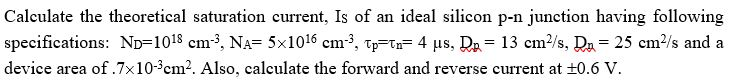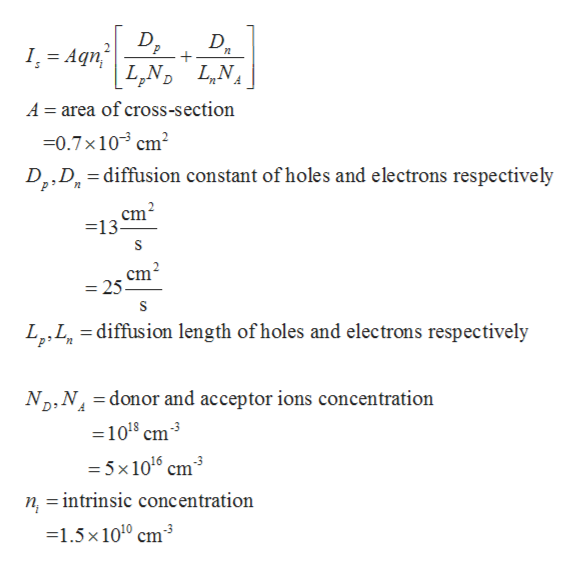# Calculate the theoretical saturation current, Is of an ideal silicon p-n junction having followingspecifications: ND 1018 cm3, NA= 5x1016 cm3, TT 4 us, D= 13 cm2/s, D 25 cm2/s and adevice area of .7x10-3cm2. Also, calculate the forward and reverse current at +0.6 V

Question
3 viewshelp_outlineImage TranscriptioncloseCalculate the theoretical saturation current, Is of an ideal silicon p-n junction having following specifications: ND 1018 cm3, NA= 5x1016 cm3, TT 4 us, D= 13 cm2/s, D 25 cm2/s and a device area of .7x10-3cm2. Also, calculate the forward and reverse current at +0.6 V fullscreen
check_circle

Step 1

Theoretical saturation current Is is calculated as,help_outlineImage TranscriptioncloseI, Aqn + A area of cross-section 0.7x 103 cm D2,D diffusion constant of holes and electrons respectively cm2 =13 cm2 =25 - diffusion length of holes and electrons respectively Np,N donor and acceptor ions concentration =108 cm3 = 5x 106 cm3 n =intrinsic concentration 1.5x 1010 cm3 fullscreen
Step 2

Putting all values in expression,

Step 3

Forward current at 0.6 V ...

### Want to see the full answer?

See Solution

#### Want to see this answer and more?

Solutions are written by subject experts who are available 24/7. Questions are typically answered within 1 hour.*

See Solution
*Response times may vary by subject and question.
Tagged in

### Electrical Engineering